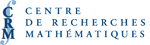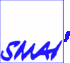# PARTENAIRESPrix Cecil Graham pour thèse de doctorat de la SCMAI

ALYSSON M. COSTA, Universidade de São Paulo
Models and algorithms for two network design problems
[PDF]

Network design problems arise in a wide variety of technological and scientific fields as a response to real-life situations and practical challenges. In this talk, we exemplify the practical aspect of these problems and present new models and algorithms for two of their variants: the Multicommodity Capacitated Fixed charge Network Design Problem (MCFNDP) and the Steiner Tree Problem with Profits (STPP).

First, we concentrate on the MCFNDP, which consists of selecting edges and flows in a graph in order to supply certain demands while respecting edge capacities. We study the interrelations between three important groups of valid inequalities for this problem: Benders, metric and cutset inequalities. Based on these relationships, we propose a simple procedure to strengthen Benders inequalities by means of quick shortest path calculations. We also develop a new Benders decomposition algorithm to solve the problem, which makes use of an original procedure for generating extra cuts.

We then present some results for the STPP, a variant of the Steiner problem in which revenues are associated with each vertex of the graph. We introduce a new variant of the problem which includes a budget constraint limiting the total network cost, and hop constraints limiting the maximum number of edges between the root vertex and any other vertex in the graph. For this variant, we first develop and compare new models and exact algorithms capable of solving mid-sized instances to optimality. We then present several heuristic procedures, including a greedy algorithm and a tabu search heuristic.